# Download PDF of RS Aggarwal Solutions for Class 10 Maths Chapter 1 Real Numbers Exercise 1B

R S Aggarwal Solutions for Class 10 Maths Chapter 1 Real Numbers, contains solutions for all Exercise 1B questions. Class 10 real numbers Exercise 1B questions and answers help students to revise on concepts such as arithmetic fundamental theorem and relationship between HCF and LCM. Download the RS Aggarwal Solutions of Class 10  and sharpen your skills.

### Download PDF of RS Aggarwal Solutions for Class 10 Maths Chapter 1 Real Numbers Exercise 1B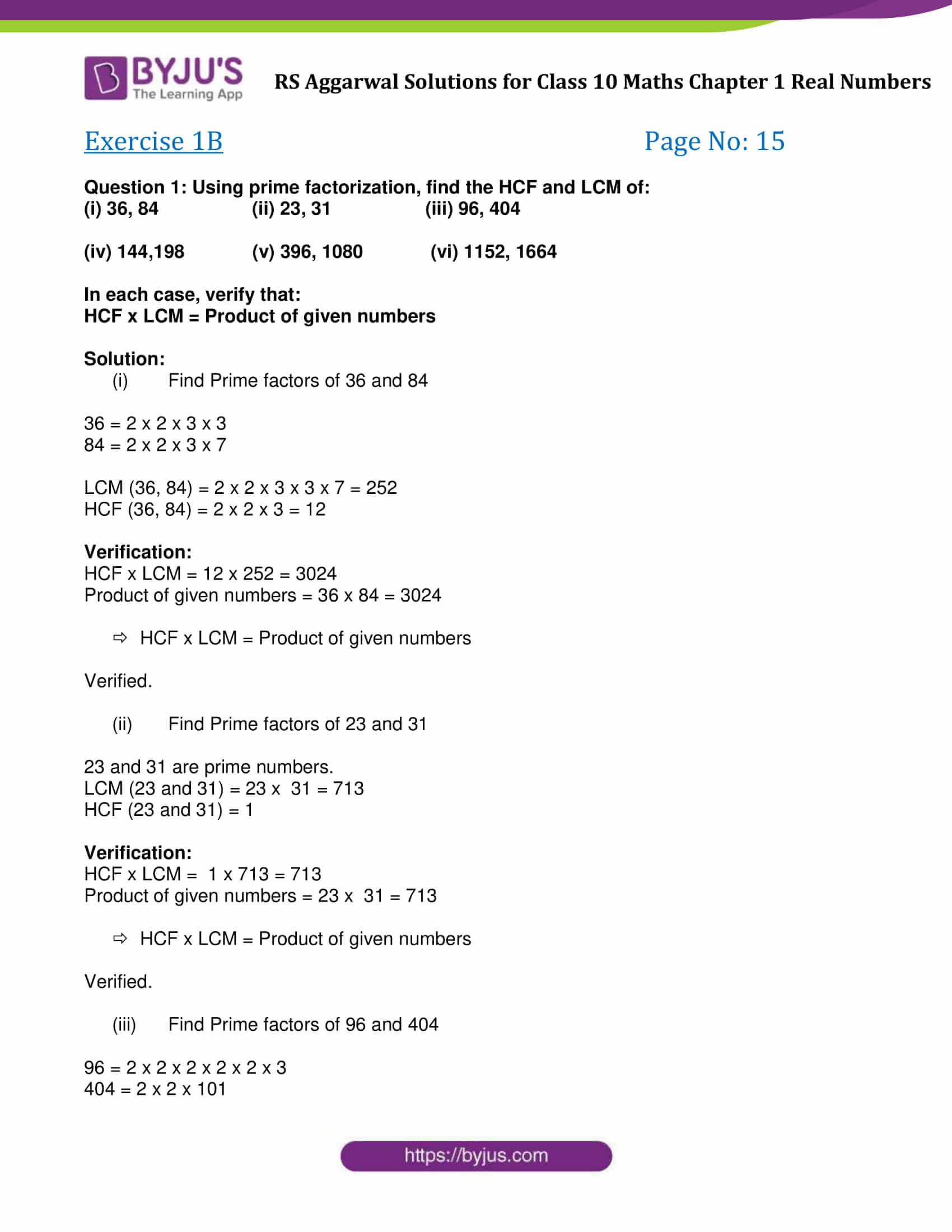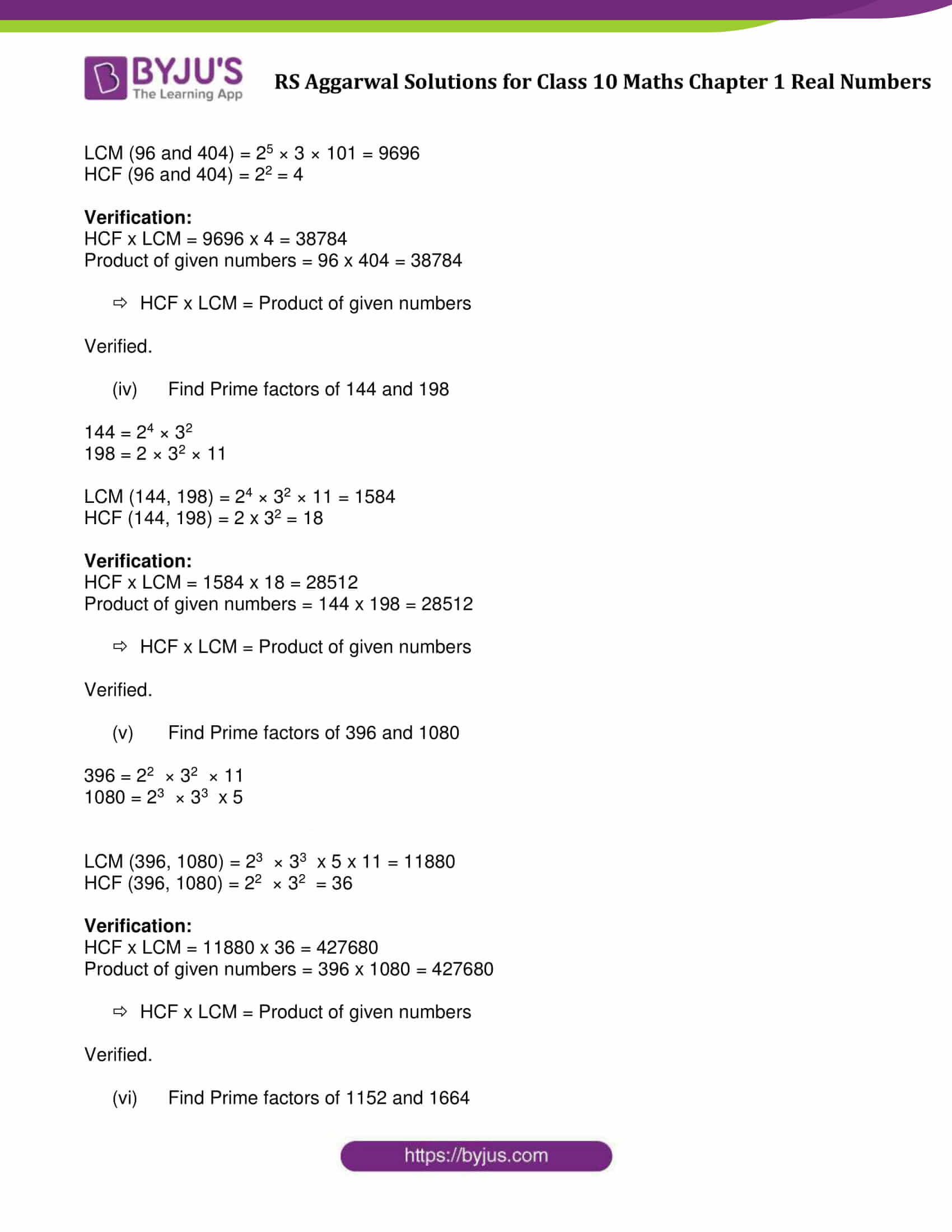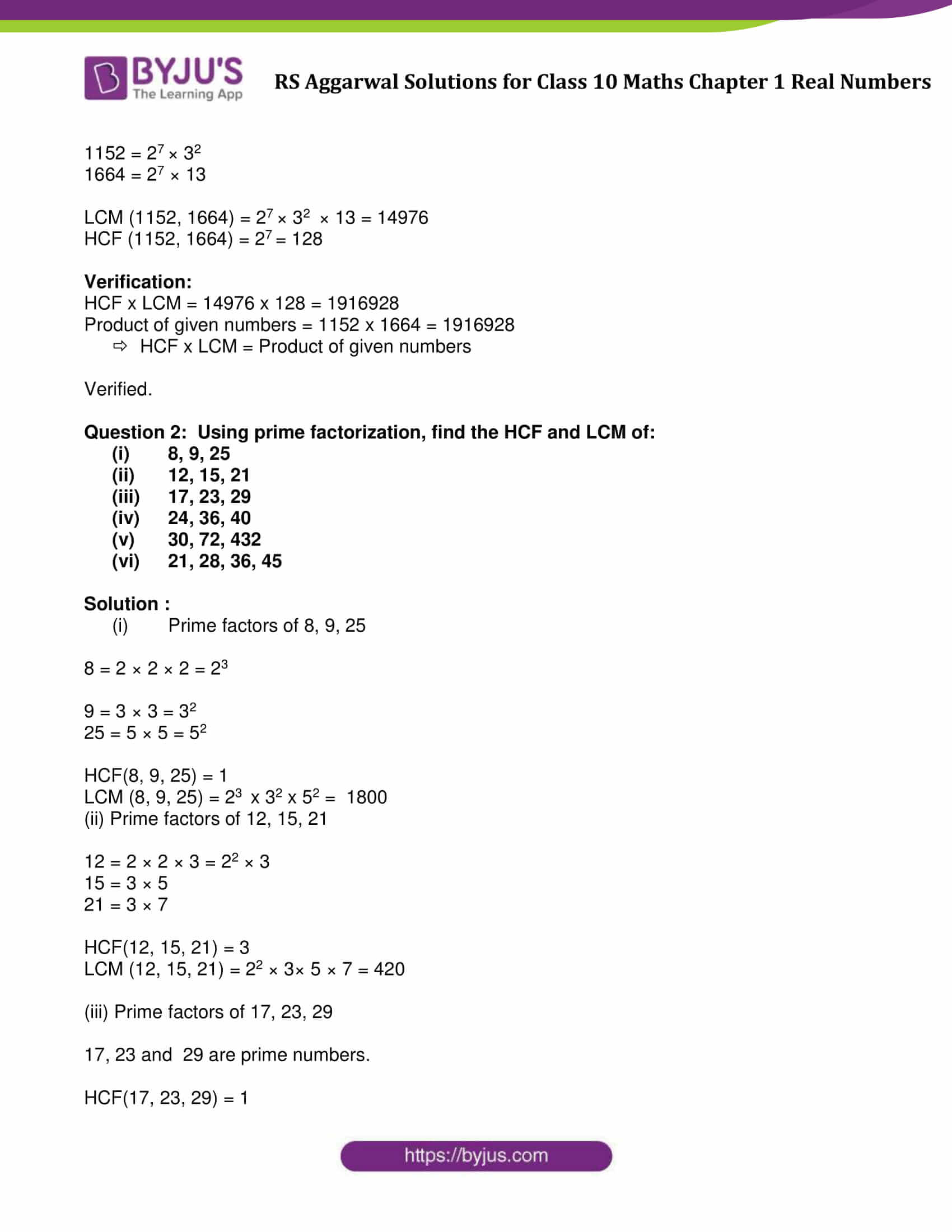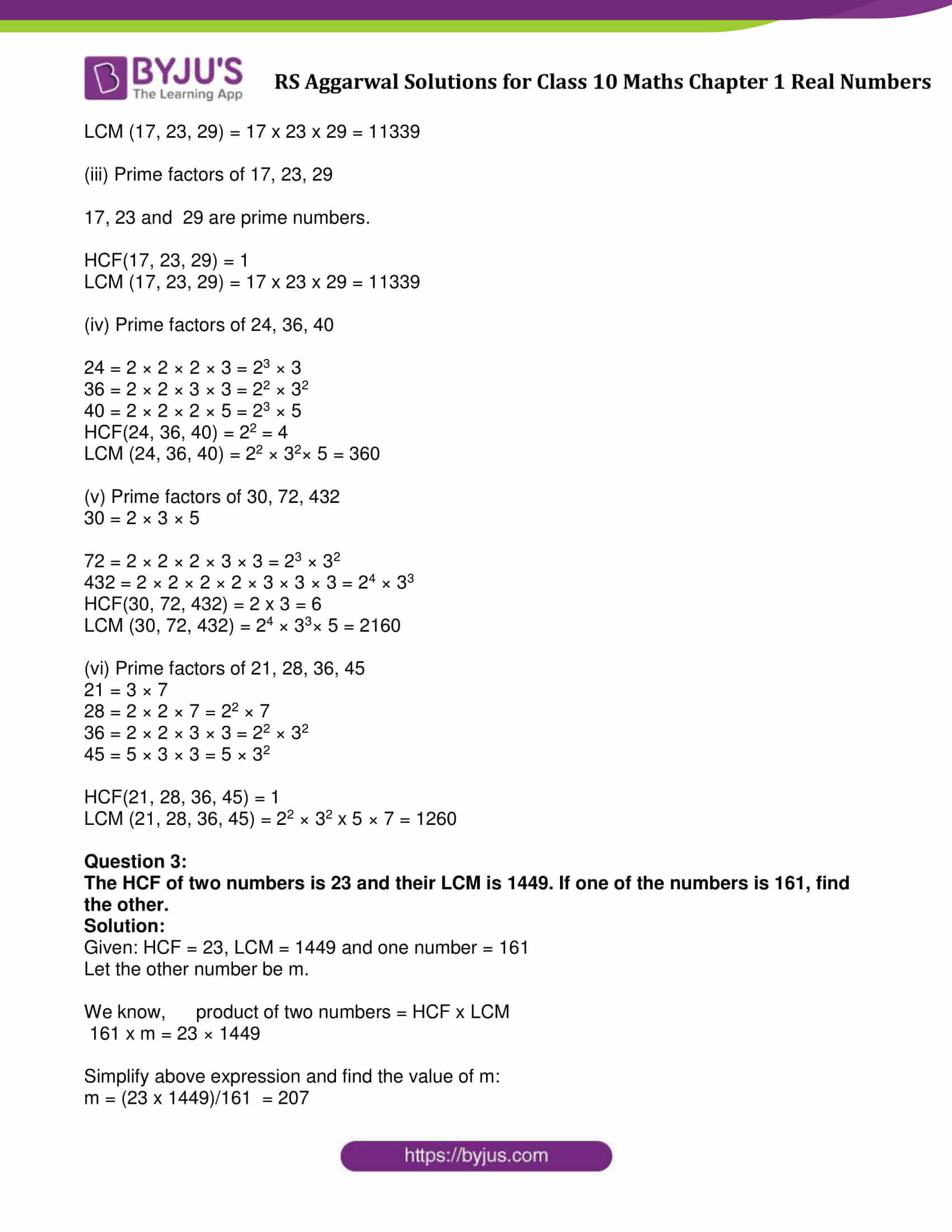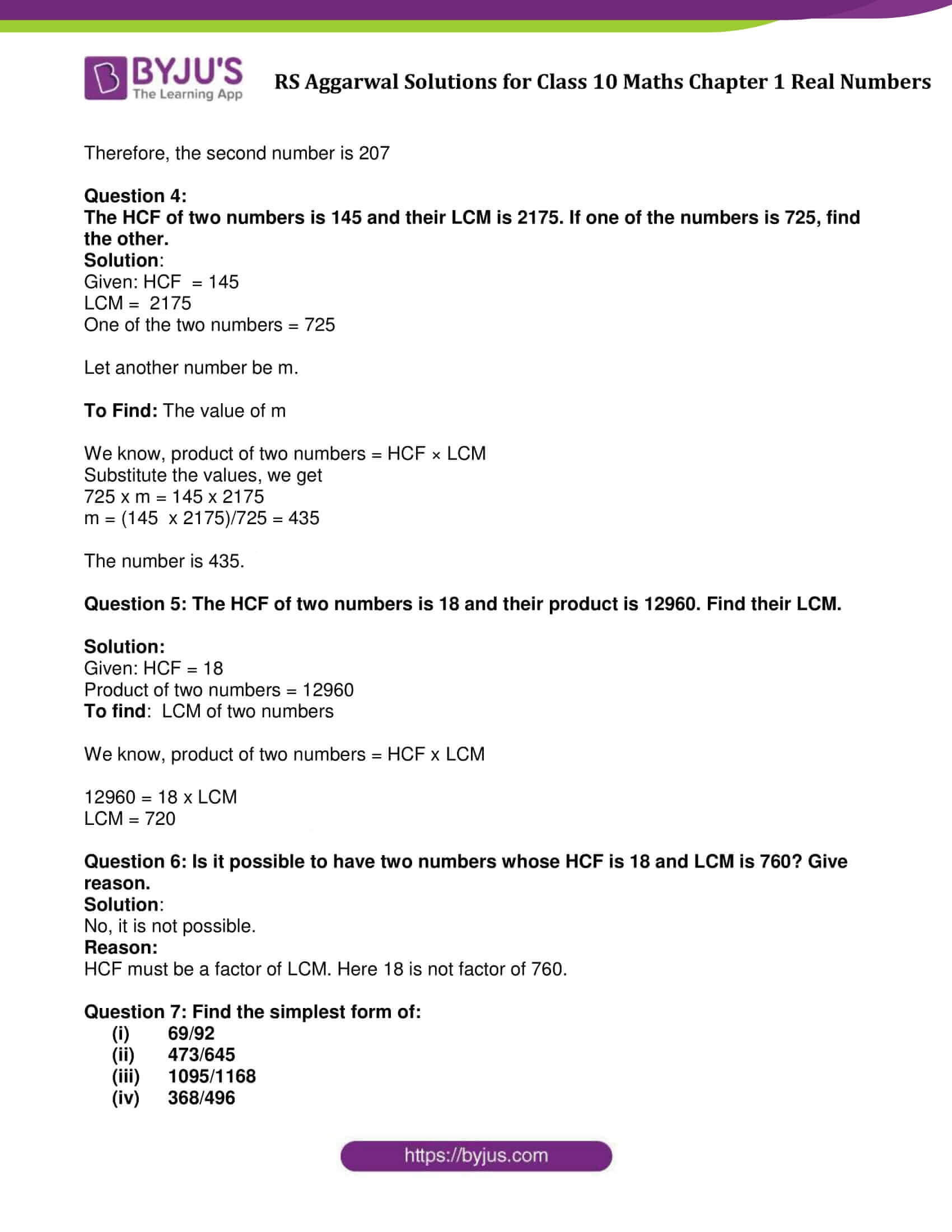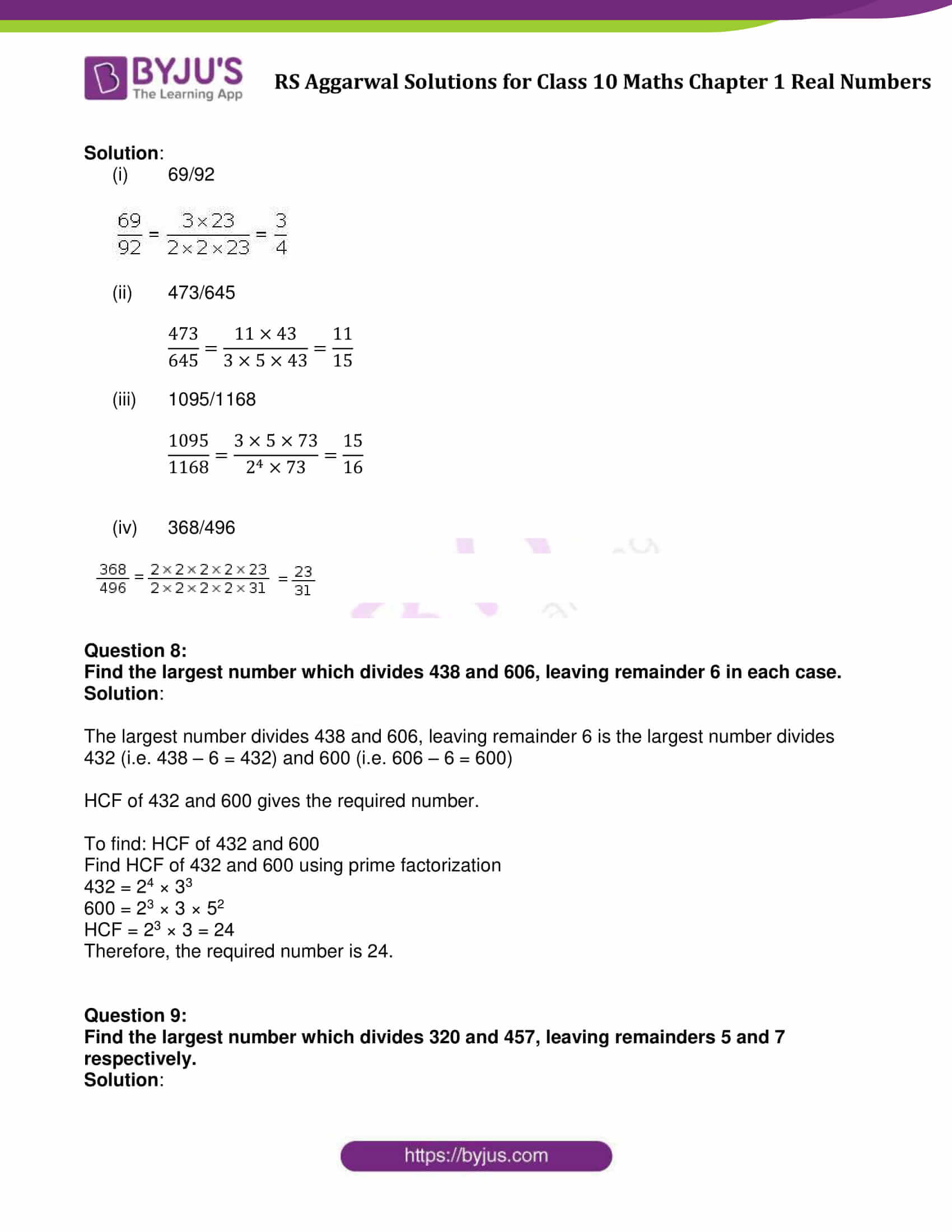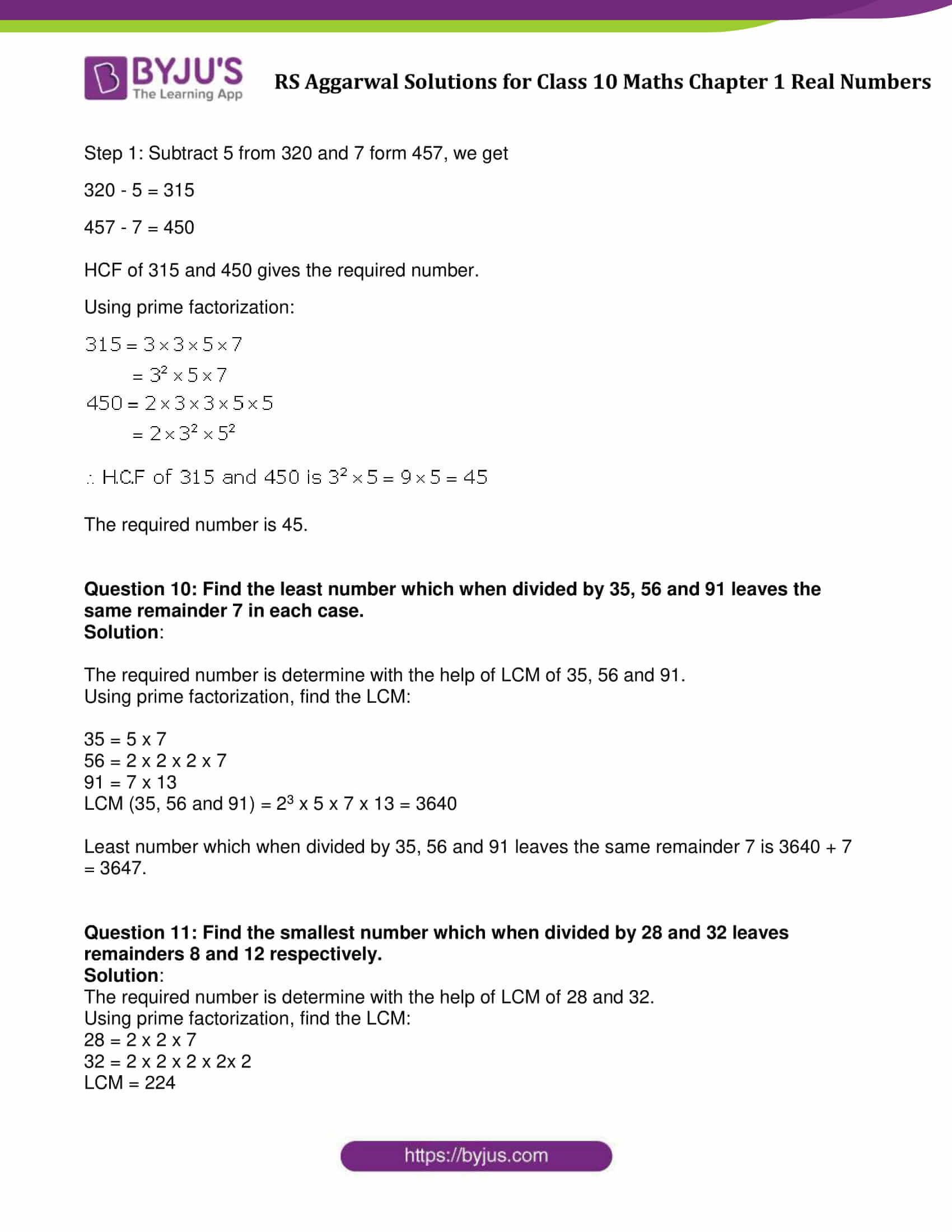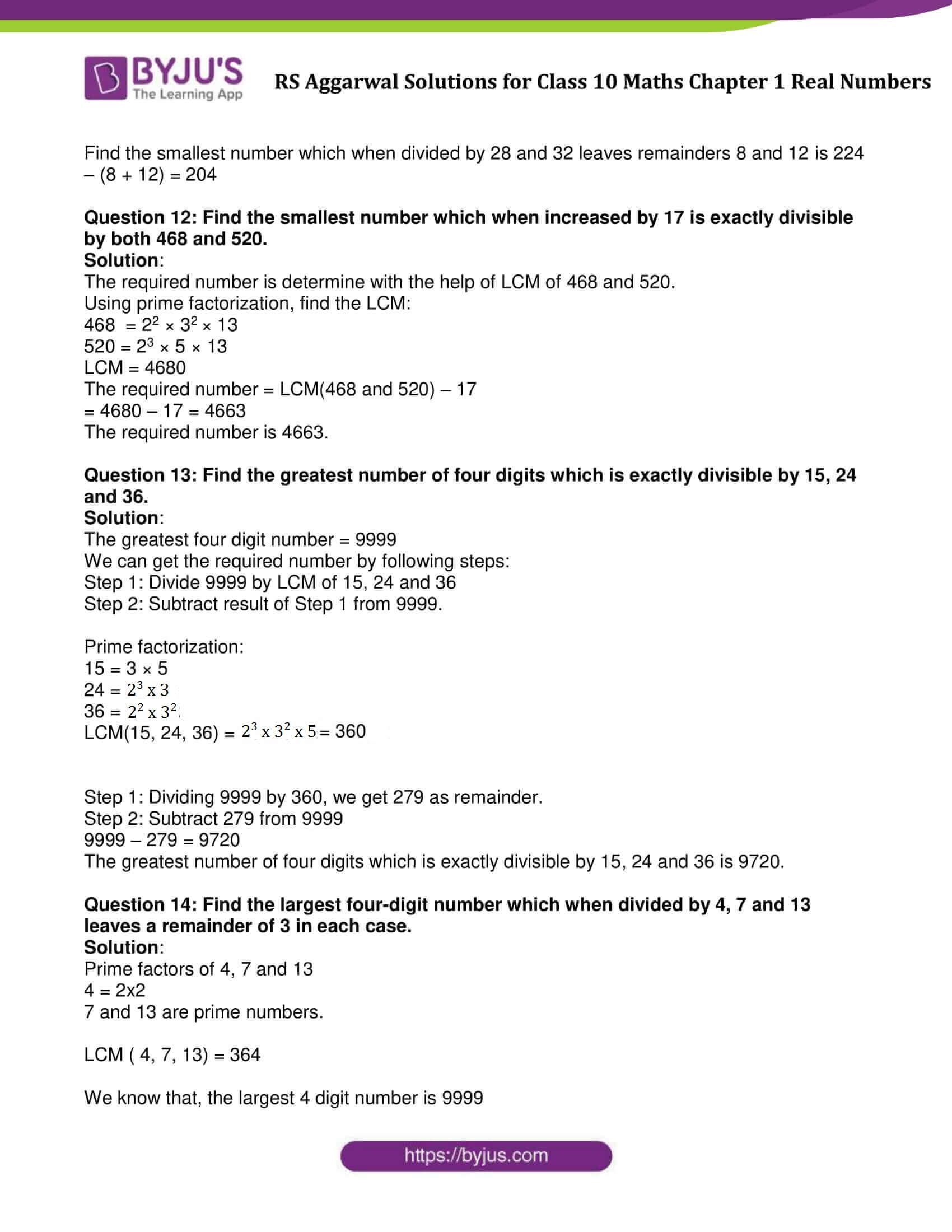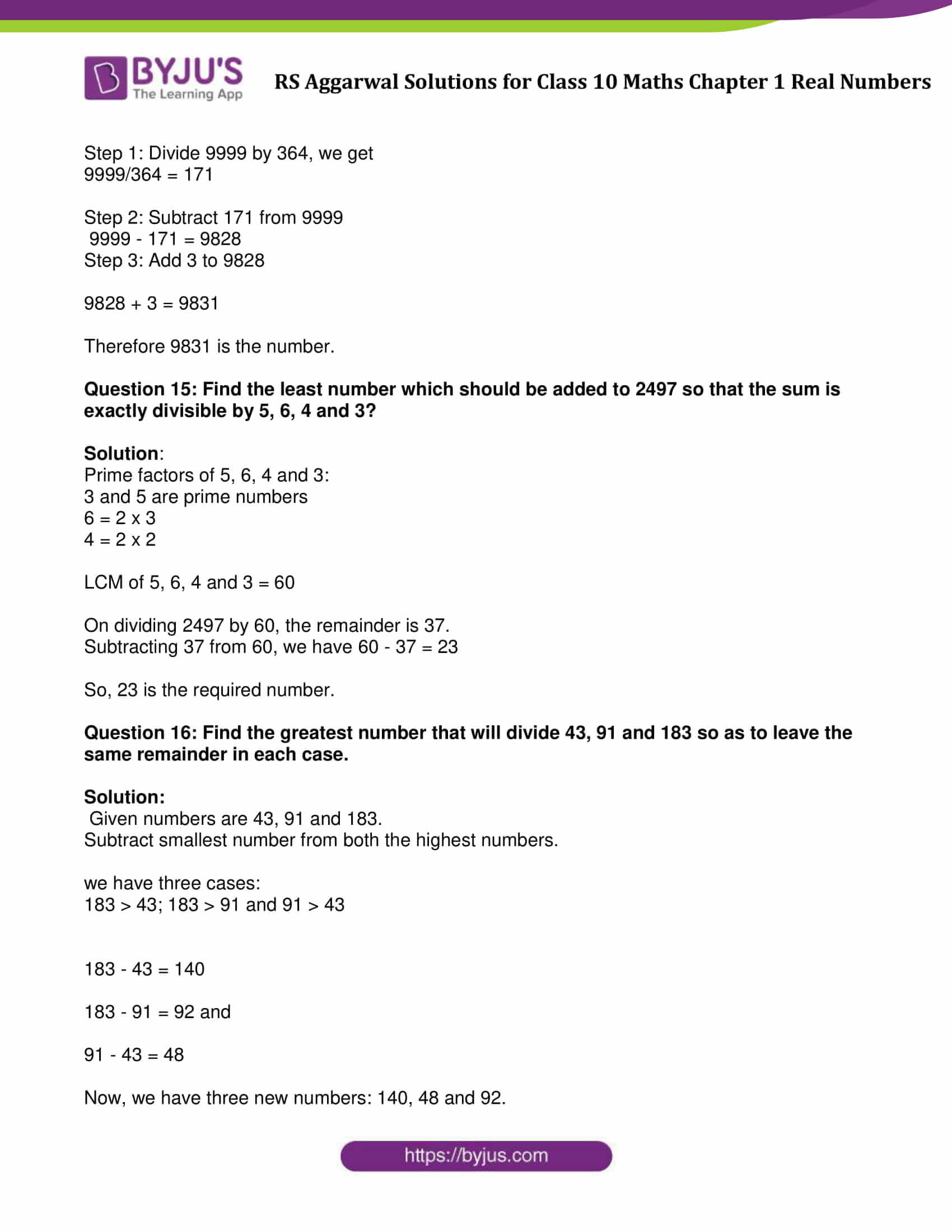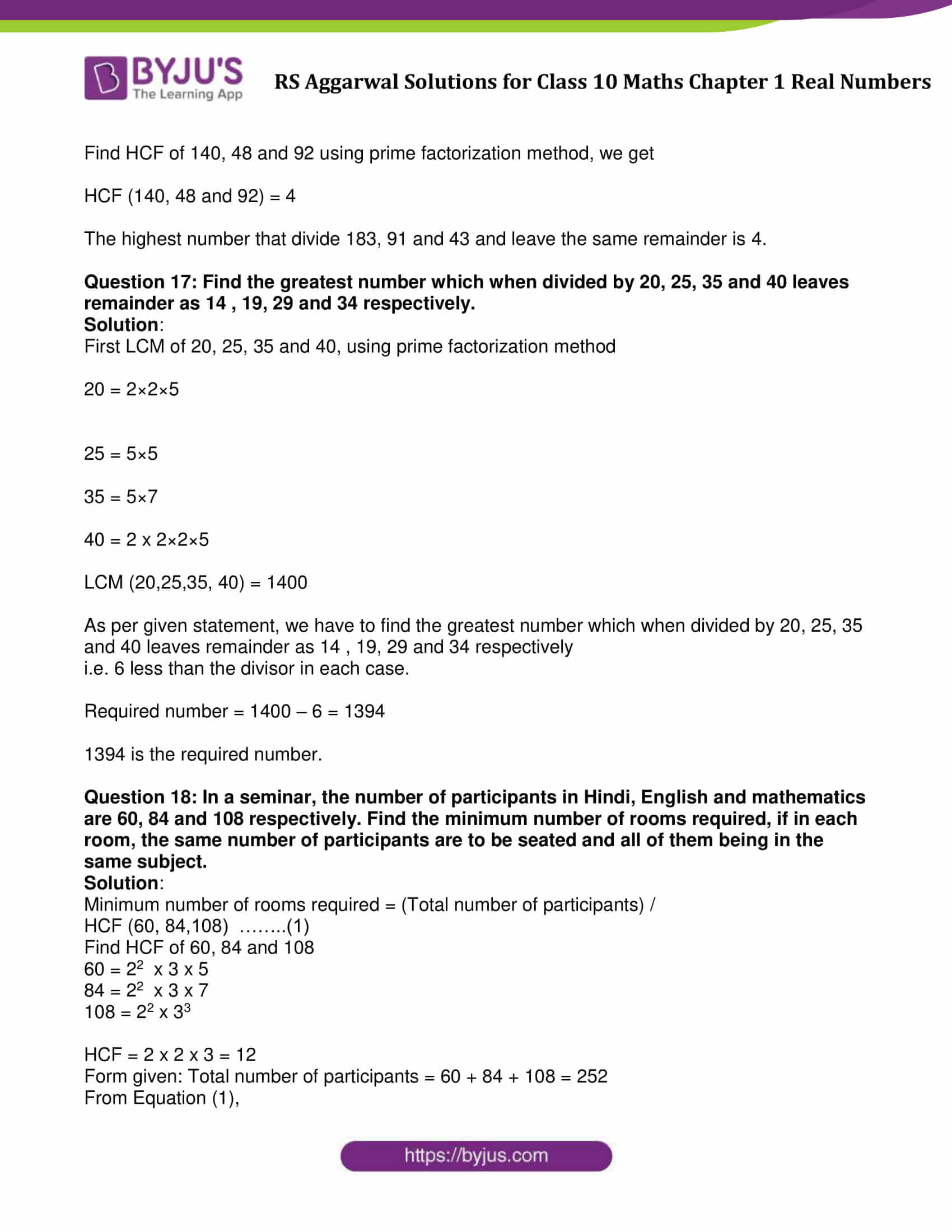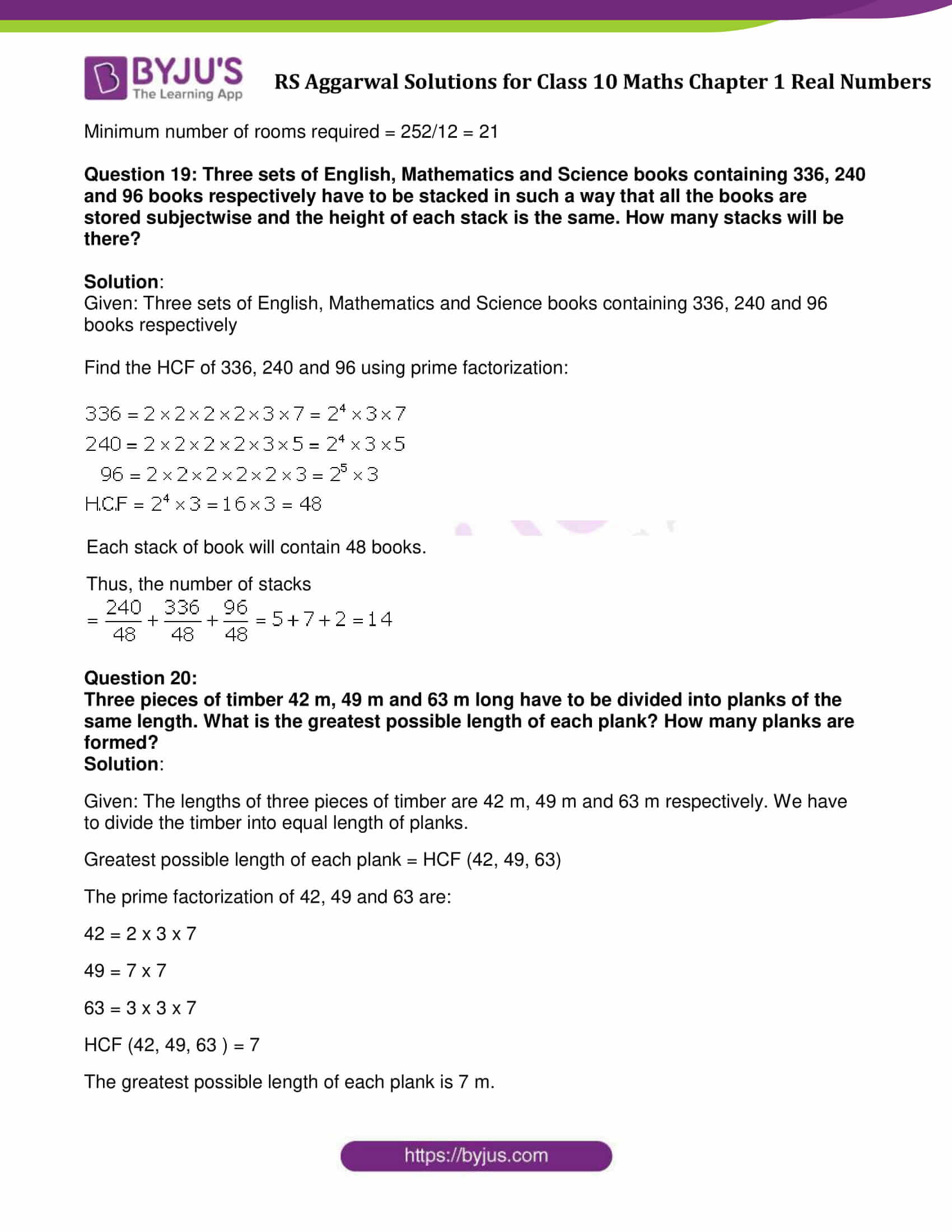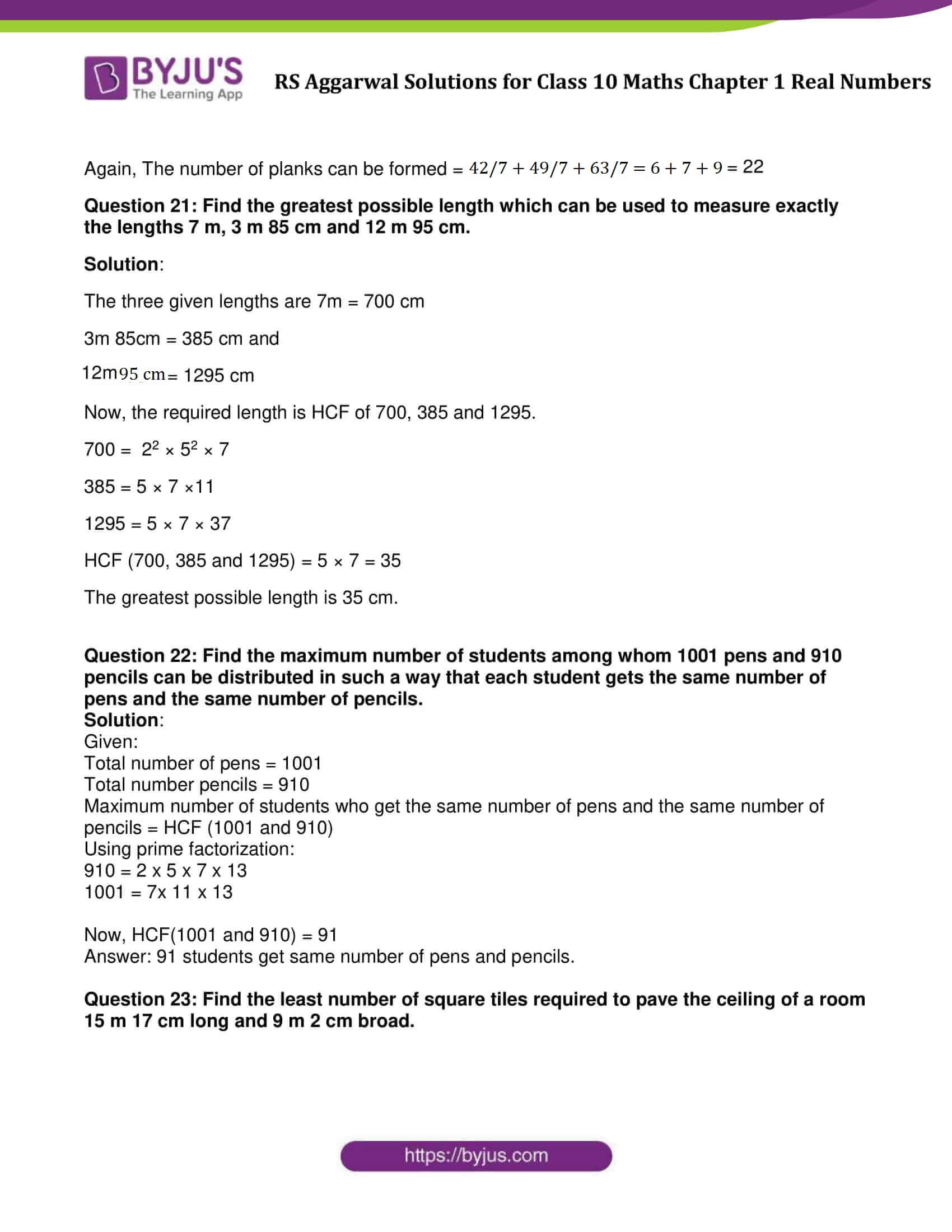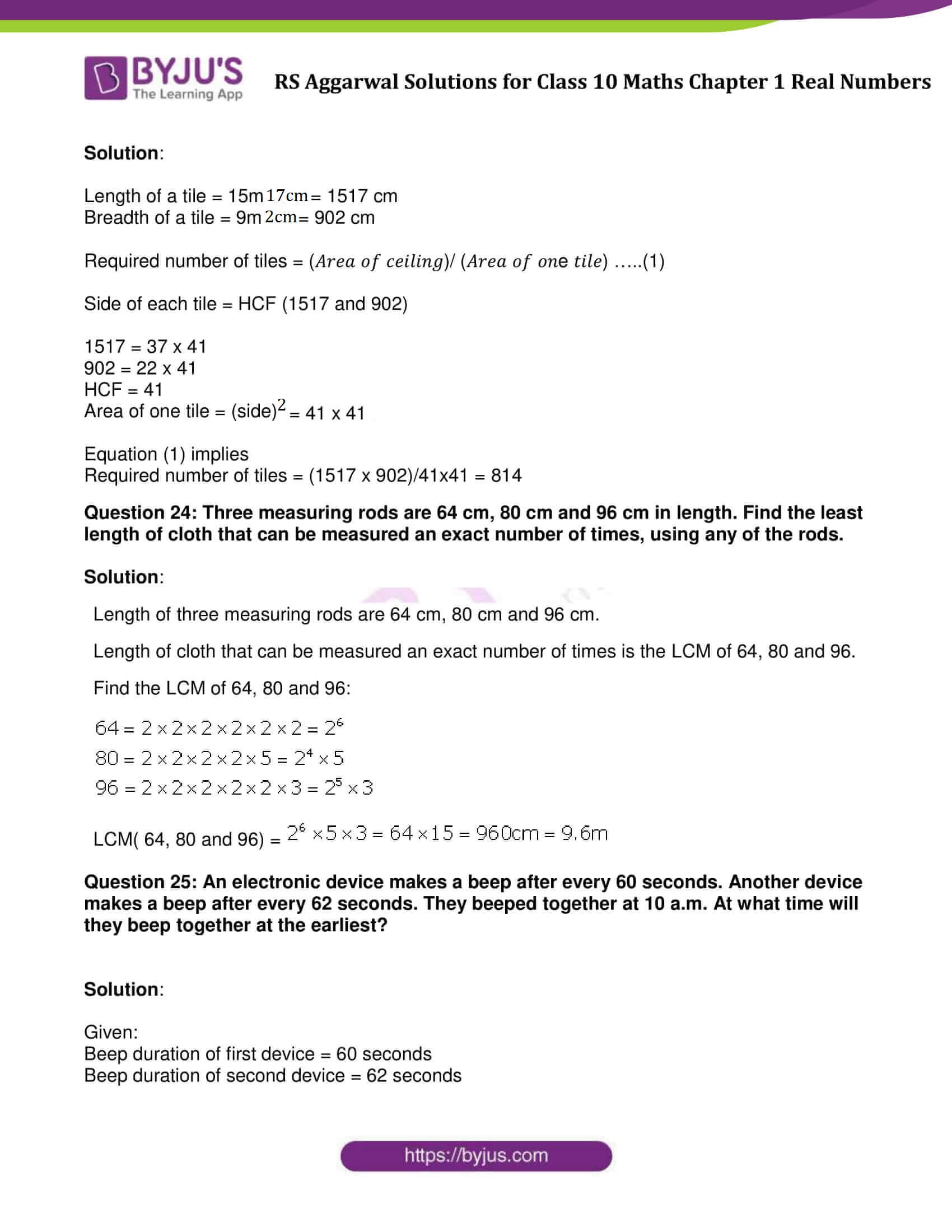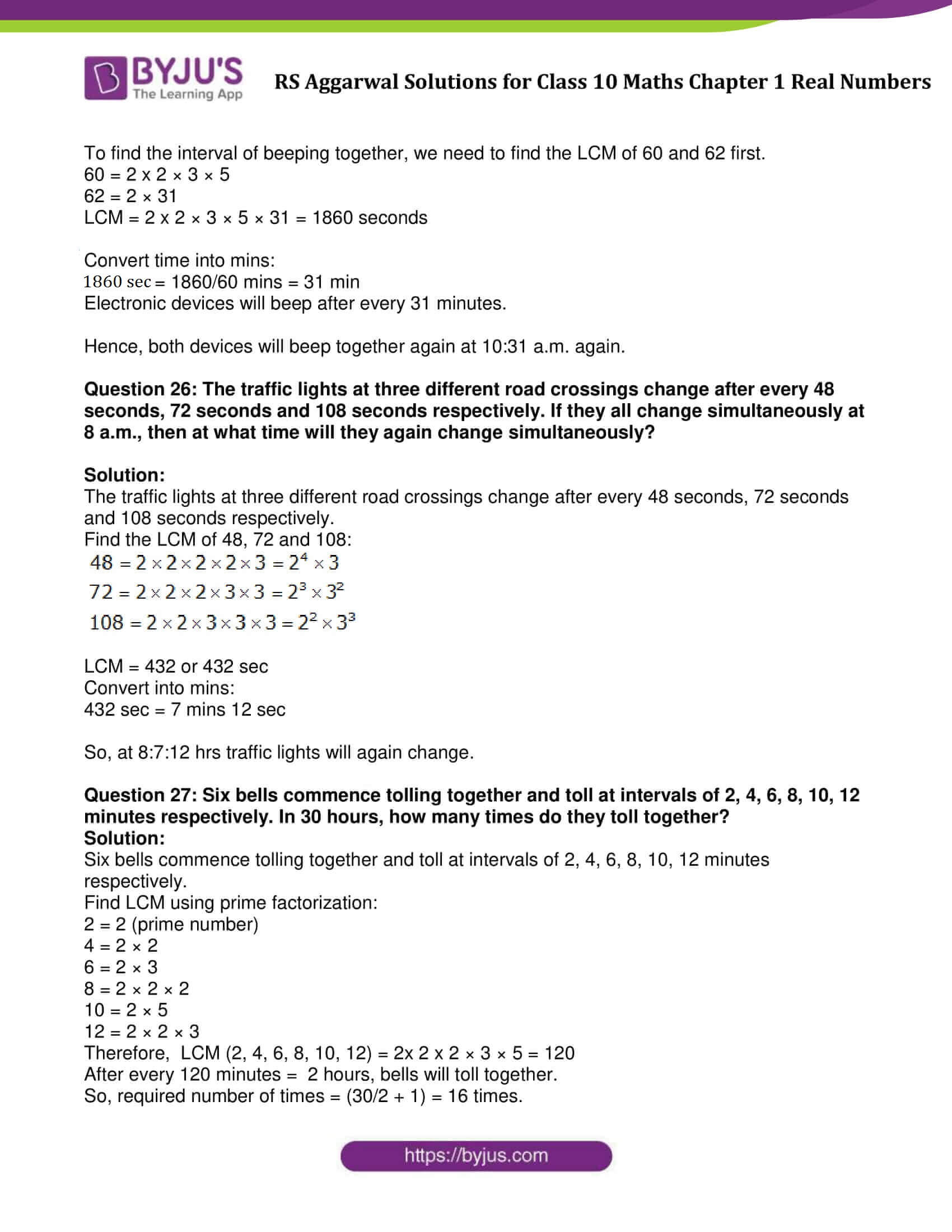### Access other exercise solutions of Class 10 Maths Chapter 1 Real Numbers

Exercise 1A Solutions : 10 Questions (Short Answers)

Exercise 1C Solutions : 3 Questions (Short Answers)

Exercise 1D Solutions : 11 Questions (Short Answers)

Exercise 1E Solutions : 23 Questions (Short Answers)

## Exercise 1B Page No: 15

Question 1: Using prime factorization, find the HCF and LCM of:

(i) 36, 84 (ii) 23, 31 (iii) 96, 404

(iv) 144,198 (v) 396, 1080 (vi) 1152, 1664

In each case, verify that:

HCF x LCM = Product of given numbers

Solution:

(i)Find Prime factors of 36 and 84

36 = 2 x 2 x 3 x 3

84 = 2 x 2 x 3 x 7

LCM (36, 84) = 2 x 2 x 3 x 3 x 7 = 252

HCF (36, 84) = 2 x 2 x 3 = 12

Verification:

HCF x LCM = 12 x 252 = 3024

Product of given numbers = 36 x 84 = 3024

⇨ HCF x LCM = Product of given numbers

Verified.

(ii)Find Prime factors of 23 and 31

23 and 31 are prime numbers.

LCM (23 and 31) = 23 x 31 = 713

HCF (23 and 31) = 1

Verification:

HCF x LCM = 1 x 713 = 713

Product of given numbers = 23 x 31 = 713

⇨ HCF x LCM = Product of given numbers

Verified.

(iii)Find Prime factors of 96 and 404

96 = 2 x 2 x 2 x 2 x 2 x 3

404 = 2 x 2 x 101

LCM (96 and 404) = 25 × 3 × 101 = 9696

HCF (96 and 404) = 22 = 4

Verification:

HCF x LCM = 9696 x 4 = 38784

Product of given numbers = 96 x 404 = 38784

⇨ HCF x LCM = Product of given numbers

Verified.

(iv) Find Prime factors of 144 and 198

144 = 24 × 32

198 = 2 × 32 × 11

LCM (144, 198) = 24 × 32 × 11 = 1584

HCF (144, 198) = 2 x 32 = 18

Verification:

HCF x LCM = 1584 x 18 = 28512

Product of given numbers = 144 x 198 = 28512

⇨ HCF x LCM = Product of given numbers

Verified.

(v)Find Prime factors of 396 and 1080

396 = 22 × 32 × 11

1080 = 23 × 33 x 5

LCM (396, 1080) = 23 × 33 x 5 x 11 = 11880

HCF (396, 1080) = 22 × 32 = 36

Verification:

HCF x LCM = 11880 x 36 = 427680

Product of given numbers = 396 x 1080 = 427680

⇨ HCF x LCM = Product of given numbers

Verified.

(vi) Find Prime factors of 1152 and 1664

1152 = 27 × 32

1664 = 27 × 13

LCM (1152, 1664) = 27 × 32 × 13 = 14976

HCF (1152, 1664) = 27 = 128

Verification:

HCF x LCM = 14976 x 128 = 1916928

Product of given numbers = 1152 x 1664 = 1916928

⇨ HCF x LCM = Product of given numbers

Verified.

Question 2: Using prime factorization, find the HCF and LCM of:

(i) 8, 9, 25
(ii) 12, 15, 21
(iii) 17, 23, 29
(iv) 24, 36, 40
(v) 30, 72, 432
(vi) 21, 28, 36, 45

Solution :

(i) Prime factors of 8, 9, 25

8 = 2 × 2 × 2 = 23

9 = 3 × 3 = 32

25 = 5 × 5 = 52

HCF(8, 9, 25) = 1

LCM (8, 9, 25) = 23 x 32 x 52 = 1800

(ii) Prime factors of 12, 15, 21

12 = 2 × 2 × 3 = 22 × 3

15 = 3 × 5

21 = 3 × 7

HCF(12, 15, 21) = 3

LCM (12, 15, 21) = 22 × 3× 5 × 7 = 420

(iii) Prime factors of 17, 23, 29

17, 23 and 29 are prime numbers.

HCF(17, 23, 29) = 1

LCM (17, 23, 29) = 17 x 23 x 29 = 11339

(iii) Prime factors of 17, 23, 29

17, 23 and 29 are prime numbers.

HCF(17, 23, 29) = 1

LCM (17, 23, 29) = 17 x 23 x 29 = 11339

(iv) Prime factors of 24, 36, 40

24 = 2 × 2 × 2 × 3 = 23 × 3

36 = 2 × 2 × 3 × 3 = 22 × 32

40 = 2 × 2 × 2 × 5 = 23 × 5

HCF(24, 36, 40) = 22 = 4

LCM (24, 36, 40) = 22 × 32× 5 = 360

(v) Prime factors of 30, 72, 432

30 = 2 × 3 × 5

72 = 2 × 2 × 2 × 3 × 3 = 23 × 32

432 = 2 × 2 × 2 × 2 × 3 × 3 × 3 = 24 × 33

HCF(30, 72, 432) = 2 x 3 = 6

LCM (30, 72, 432) = 24 × 33× 5 = 2160

(vi) Prime factors of 21, 28, 36, 45

21 = 3 × 7

28 = 2 × 2 × 7 = 22 × 7

36 = 2 × 2 × 3 × 3 = 22 × 32

45 = 5 × 3 × 3 = 5 × 32

HCF(21, 28, 36, 45) = 1

LCM (21, 28, 36, 45) = 22 × 32 x 5 × 7 = 1260

Question 3: The HCF of two numbers is 23 and their LCM is 1449. If one of the numbers is 161, find the other.

Solution:

Given: HCF = 23, LCM = 1449 and one number = 161

Let the other number be m.

We know, product of two numbers = HCF x LCM

161 x m = 23 × 1449

Simplify above expression and find the value of m:

m = (23 x 1449)/161 = 207

Therefore, the second number is 207

Question 4:

The HCF of two numbers is 145 and their LCM is 2175. If one of the numbers is 725, find the other.

Solution:

Given: HCF = 145

LCM = 2175

One of the two numbers = 725

Let another number be m.

To Find: The value of m

We know, product of two numbers = HCF × LCM

Substitute the values, we get

725 x m = 145 x 2175

m = (145 x 2175)/725 = 435

The number is 435.

Question 5: The HCF of two numbers is 18 and their product is 12960. Find their LCM.

Solution:

Given: HCF = 18

Product of two numbers = 12960

To find: LCM of two numbers

We know, product of two numbers = HCF x LCM

12960 = 18 x LCM

LCM = 720

Question 6: Is it possible to have two numbers whose HCF is 18 and LCM is 760? Give reason.

Solution:

No, it is not possible.

Reason:

HCF must be a factor of LCM. Here 18 is not factor of 760.

Question 7: Find the simplest form of:

(i) 69/92
(ii) 473/645
(iii) 1095/1168
(iv) 368/496

Solution:

(i) 69/92
$\frac{69}{92} = \frac{3\times23}{2\times2\times23} = \frac{3}{4}$

(ii) 473/645
$\frac{473}{645} = \frac{11\times43}{3\times5\times43} = \frac{11}{15}$

(iii) 1095/1168
$\frac{1095}{1168} = \frac{3\times5\times73}{2^{4}\times73} = \frac{15}{16}$

(iv) 368/496
$\frac{368}{496} = \frac{2\times2\times2\times2\times23}{2\times2\times2\times2\times31} = \frac{23}{31}$

Question 8:

Find the largest number which divides 438 and 606, leaving remainder 6 in each case.

Solution:

The largest number divides 438 and 606, leaving remainder 6 is the largest number divides 432 (i.e. 438 – 6 = 432) and 600 (i.e. 606 – 6 = 600)

HCF of 432 and 600 gives the required number.

To find: HCF of 432 and 600

Find HCF of 432 and 600 using prime factorization

432 = 24 × 33

600 = 23 × 3 × 52

HCF = 23 × 3 = 24

Therefore, the required number is 24.

Question 9:

Find the largest number which divides 320 and 457, leaving remainders 5 and 7 respectively.

Solution:

Step 1: Subtract 5 from 320 and 7 form 457, we get

320 – 5 = 315

457 – 7 = 450

HCF of 315 and 450 gives the required number.

Using prime factorization:

315 = 3 × 3 × 5 × 7

= 32 × 5 × 7

450 = 2 × 3 × 3 × 5 × 5

= 2 × 32 × 52

HCF of 315 and 450 is 32× 5 = 9 × 5 = 45

The required number is 45.

Question 10: Find the least number which when divided by 35, 56 and 91 leaves the same remainder 7 in each case.

Solution:

The required number is determine with the help of LCM of 35, 56 and 91.

Using prime factorization, find the LCM:

35 = 5 x 7

56 = 2 x 2 x 2 x 7

91 = 7 x 13

LCM (35, 56 and 91) = 23 x 5 x 7 x 13 = 3640

Least number which when divided by 35, 56 and 91 leaves the same remainder 7 is 3640 + 7 = 3647.

Question 11: Find the smallest number which when divided by 28 and 32 leaves remainders 8 and 12 respectively.

Solution:

The required number is determine with the help of LCM of 28 and 32.

Using prime factorization, find the LCM:

28 = 2 x 2 x 7

32 = 2 x 2 x 2 x 2x 2

LCM = 224

Find the smallest number which when divided by 28 and 32 leaves remainders 8 and 12 is 224 – (8 + 12) = 204

Question 12: Find the smallest number which when increased by 17 is exactly divisible by both 468 and 520.

Solution:

The required number is determine with the help of LCM of 468 and 520.

Using prime factorization, find the LCM:

468 = 22 × 32 × 13

520 = 23 × 5 × 13

LCM = 4680

The required number = LCM(468 and 520) – 17

= 4680 – 17 = 4663

The required number is 4663.

Question 13: Find the greatest number of four digits which is exactly divisible by 15, 24 and 36.

Solution:

The greatest four digit number = 9999

We can get the required number by following steps:

Step 1: Divide 9999 by LCM of 15, 24 and 36

Step 2: Subtract result of Step 1 from 9999.

Prime factorization:

15 = 3 × 5

24 = 23 × 3

36 = 22 × 32

LCM(15, 24, 36) = 23 × 32 × 5 = 360

Step 1: Dividing 9999 by 360, we get 279 as remainder.

Step 2: Subtract 279 from 9999

9999 – 279 = 9720

The greatest number of four digits which is exactly divisible by 15, 24 and 36 is 9720.

Question 14: Find the largest four-digit number which when divided by 4, 7 and 13 leaves a remainder of 3 in each case.

Solution:

Prime factors of 4, 7 and 13

4 = 2×2

7 and 13 are prime numbers.

LCM ( 4, 7, 13) = 364

We know that, the largest 4 digit number is 9999

Step 1: Divide 9999 by 364, we get

9999/364 = 171

Step 2: Subtract 171 from 9999

9999 – 171 = 9828

Step 3: Add 3 to 9828

9828 + 3 = 9831

Therefore 9831 is the number.

Question 15: Find the least number which should be added to 2497 so that the sum is exactly divisible by 5, 6, 4 and 3?

Solution:

Prime factors of 5, 6, 4 and 3:

3 and 5 are prime numbers

6 = 2 x 3

4 = 2 x 2

LCM of 5, 6, 4 and 3 = 60

On dividing 2497 by 60, the remainder is 37.

Subtracting 37 from 60, we have 60 – 37 = 23

So, 23 is the required number.

Question 16: Find the greatest number that will divide 43, 91 and 183 so as to leave the same remainder in each case.

Solution:

Given numbers are 43, 91 and 183.

Subtract smallest number from both the highest numbers.

we have three cases:

183 > 43; 183 > 91 and 91 > 43

183 – 43 = 140

183 – 91 = 92 and

91 – 43 = 48

Now, we have three new numbers: 140, 48 and 92.

Find HCF of 140, 48 and 92 using prime factorization method, we get

HCF (140, 48 and 92) = 4

The highest number that divide 183, 91 and 43 and leave the same remainder is 4.

Question 17: Find the greatest number which when divided by 20, 25, 35 and 40 leaves remainder as 14 , 19, 29 and 34 respectively.

Solution:

First LCM of 20, 25, 35 and 40, using prime factorization method

20 = 2×2×5

25 = 5×5

35 = 5×7

40 = 2 x 2×2×5

LCM (20,25,35, 40) = 1400

As per given statement, we have to find the greatest number which when divided by 20, 25, 35 and 40 leaves remainder as 14 , 19, 29 and 34 respectively

i.e. 6 less than the divisor in each case.

Required number = 1400 – 6 = 1394

1394 is the required number.

Question 18: In a seminar, the number of participants in Hindi, English and mathematics are 60, 84 and 108 respectively. Find the minimum number of rooms required, if in each room, the same number of participants are to be seated and all of them being in the same subject.

Solution:

Minimum number of rooms required = (Total number of participants) /

HCF (60, 84,108) ……..(1)

Find HCF of 60, 84 and 108

60 = 22 x 3 x 5

84 = 22 x 3 x 7

108 = 22 x 33

HCF = 2 x 2 x 3 = 12

Form given: Total number of participants = 60 + 84 + 108 = 252

From Equation (1),

Minimum number of rooms required = 252/12 = 21

Question 19: Three sets of English, Mathematics and Science books containing 336, 240 and 96 books respectively have to be stacked in such a way that all the books are stored subjectwise and the height of each stack is the same. How many stacks will be there?

Solution:

Given: Three sets of English, Mathematics and Science books containing 336, 240 and 96 books respectively

Find the HCF of 336, 240 and 96 using prime factorization:

336 = 2 × 2 × 2 × 2 × 3 × 7 = 24 × 3 × 7

240 = 2 × 2 × 2 × 2 × 3 × 4 = 24 × 3 × 5

96 = 2 × 2 × 2 × 2 × 2 × 3 = 25 × 3

HCF = 24 × 3 = 16 × 3 = 48

Each stack of book will contain 48 books.

Thus, the number of stacks

$\frac{240}{48} + \frac{336}{48} + \frac{96}{48} = 5 + 7 +2 = 14$

Question 20:

Three pieces of timber 42 m, 49 m and 63 m long have to be divided into planks of the same length. What is the greatest possible length of each plank? How many planks are formed?

Solution:

Given: The lengths of three pieces of timber are 42 m, 49 m and 63 m respectively. We have to divide the timber into equal length of planks.

Greatest possible length of each plank = HCF (42, 49, 63)

The prime factorization of 42, 49 and 63 are:

42 = 2 x 3 x 7

49 = 7 x 7

63 = 3 x 3 x 7

HCF (42, 49, 63 ) = 7

The greatest possible length of each plank is 7 m.

Again, The number of planks can be formed = 42/7 + 49/7 + 63/7 = 6 + 7 + 9 = 22

Question 21: Find the greatest possible length which can be used to measure exactly the lengths 7 m, 3 m 85 cm and 12 m 95 cm.

Solution:

The three given lengths are 7m = 700 cm

3m 85cm = 385 cm and

12m 95cm = 1295 cm

Now, the required length is HCF of 700, 385 and 1295.

700 = 22 × 52 × 7

385 = 5 × 7 ×11

1295 = 5 × 7 × 37

HCF (700, 385 and 1295) = 5 × 7 = 35

The greatest possible length is 35 cm.

Question 22: Find the maximum number of students among whom 1001 pens and 910 pencils can be distributed in such a way that each student gets the same number of pens and the same number of pencils.

Solution:

Given:

Total number of pens = 1001

Total number pencils = 910

Maximum number of students who get the same number of pens and the same number of pencils = HCF (1001 and 910)

Using prime factorization:

910 = 2 x 5 x 7 x 13

1001 = 7x 11 x 13

Now, HCF(1001 and 910) = 91

Answer: 91 students get same number of pens and pencils.

Question 23: Find the least number of square tiles required to pave the ceiling of a room 15 m 17 cm long and 9 m 2 cm broad.

Solution:

Length of a tile = 15m 17cm = 1517 cm

Breadth of a tile = 9m 2cm = 902 cm

Required number of tiles = (𝐴𝑟𝑒𝑎 𝑜𝑓 𝑐𝑒𝑖𝑙𝑖𝑛𝑔)/ (𝐴𝑟𝑒𝑎 𝑜𝑓 𝑜𝑛e 𝑡𝑖𝑙𝑒) …..(1)

Side of each tile = HCF (1517 and 902)

1517 = 37 x 41

902 = 22 x 41

HCF = 41

Area of one tile = (side)2 = 41 x 41

Equation (1) implies

Required number of tiles = (1517 x 902)/41×41 = 814

Question 24: Three measuring rods are 64 cm, 80 cm and 96 cm in length. Find the least length of cloth that can be measured an exact number of times, using any of the rods.

Solution:

Length of three measuring rods are 64 cm, 80 cm and 96 cm.

Length of cloth that can be measured an exact number of times is the LCM of 64, 80 and 96.

Find the LCM of 64, 80 and 96:

64 = 2 × 2 × 2 × 2 × 2 × 2 = 26

80 = 2 × 2 × 2 × 2 × 2 × 5 = 24

96 = 2 × 2 × 2 × 2 × 2 × 3 = 25 × 3

LCM (64,80 and 96) = 26 × 5 × 3 = 64 × 15 = 960cm = 9.6cm

Question 25: An electronic device makes a beep after every 60 seconds. Another device makes a beep after every 62 seconds. They beeped together at 10 a.m. At what time will they beep together at the earliest?

Solution:

Given:

Beep duration of first device = 60 seconds

Beep duration of second device = 62 seconds

To find the interval of beeping together, we need to find the LCM of 60 and 62 first.

60 = 2 x 2 × 3 × 5

62 = 2 × 31

LCM = 2 x 2 × 3 × 5 × 31 = 1860 seconds

Convert time into mins:

1860 sec = 1860/60 mins = 31 mins

Electronic devices will beep after every 31 minutes.

Hence, both devices will beep together again at 10:31 a.m. again.

Question 26: The traffic lights at three different road crossings change after every 48 seconds, 72 seconds and 108 seconds respectively. If they all change simultaneously at 8 a.m., then at what time will they again change simultaneously?

Solution:

The traffic lights at three different road crossings change after every 48 seconds, 72 seconds and 108 seconds respectively.

Find the LCM of 48, 72 and 108:

48 = 2 × 2 × 2 × 2 × 3 = 24 × 3

72 = 2 × 2 × 2 × 3 × 3 = 23 × 32

108 = 2 × 2 × 3 × 3 × 3= 22 × 3 3

LCM = 432 or 432 sec

Convert into mins:

432 sec = 7 mins 12 sec

So, at 8:7:12 hrs traffic lights will again change.

Question 27: Six bells commence tolling together and toll at intervals of 2, 4, 6, 8, 10, 12 minutes respectively. In 30 hours, how many times do they toll together?

Solution:

Six bells commence tolling together and toll at intervals of 2, 4, 6, 8, 10, 12 minutes respectively.

Find LCM using prime factorization:

2 = 2 (prime number)

4 = 2 × 2

6 = 2 × 3

8 = 2 × 2 × 2

10 = 2 × 5

12 = 2 × 2 × 3

Therefore, LCM (2, 4, 6, 8, 10, 12) = 2x 2 x 2 × 3 × 5 = 120

After every 120 minutes = 2 hours, bells will toll together.

So, required number of times = (30/2 + 1) = 16 times.

## RS Aggarwal Solutions for Class 10 Maths Chapter 1 Real Numbers Exercise 1B

Class 10 Maths Chapter 1 Real Numbers Exercise 1B, based on the following topics and subtopics:

• Fundamental Theorem of Arithmetic
• An Important property: Product of two given numbers = Product of their HCF and LCM